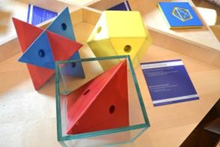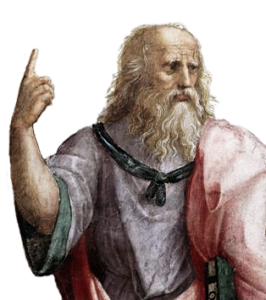# What Fits in a CubeEach solid fits perfectly in the glass cube. Can you fit the red tetrahedron in the cube? Hint: How many edges does the tetrahedron have? Can you fit the blue Kepler Star and the yellow cubeoctahedron in the cube? Hint: Examine features such as edges, faces and corners and relate those to the cube. What percentage of the cube’s volume is occupied by each solid?
Maths

Notice the tetrahedron has six edges and that a cube has six faces. This is not just a coincidence, but a geometric relationship between the tetrahedron and the cube. When you slide the tetrahedron into the cube there will be exactly one edge on each face of the cube.
When you slide the tetrahedron into the cube, the four corners of the tetrahedron occupies four corners of the cube – leaving the other four corners free. The Kepler Star is made by intersecting two tetrahedrons, so it has eight corners that can occupy all the corners of the cube.
Finally, the cubeoctahedron is made from eight triangle faces and six square faces. When you slide the solid into the glass cube, each square face will meet each face of the cube. In fact, the cubeoctahedron is made by snipping off the corners of a cube.

History

The tetrahedron is called a Platonic solid. These solids have identical regular faces (like, triangles, squares and pentagons), and the same number of faces at each corner. There are only five Platonic solids, the tetrahedron, cube, octahedron, icosahedron and dodecahedron. They are named after the ancient Greek philosopher Plato, who associated these solids with the classical elements of fire, earth, air, water and “quintessence”.
The Kepler Star is named after the famous astronomer Johannes Kepler, although he called it a stella octangular or octagonal star.
The cubeoctahedron is called an Archimedean solid, which are made with two or more types of regular faces, with the same number of faces at each corner. There are 13 Archimedean solids.

PeoplePlato c. 428BC – 348BC Plato was a philosopher from Ancient Greece. He was a student of Socrates and the teacher of Aristotle. Plato set up the first institution of higher learning in the Western world, called the Academy. While not a mathematician himself, Plato associated the five regular solids (tetrahedron, cube, octahedron, icosahedron, dodecahedron) with the four classical element (fire, earth, air, water) and a fifth element, quintessence.
Applications
Maths at Home